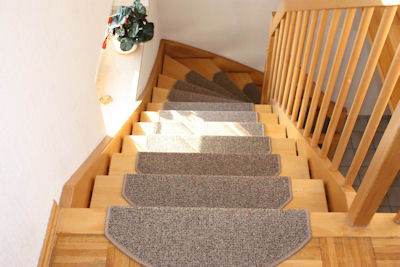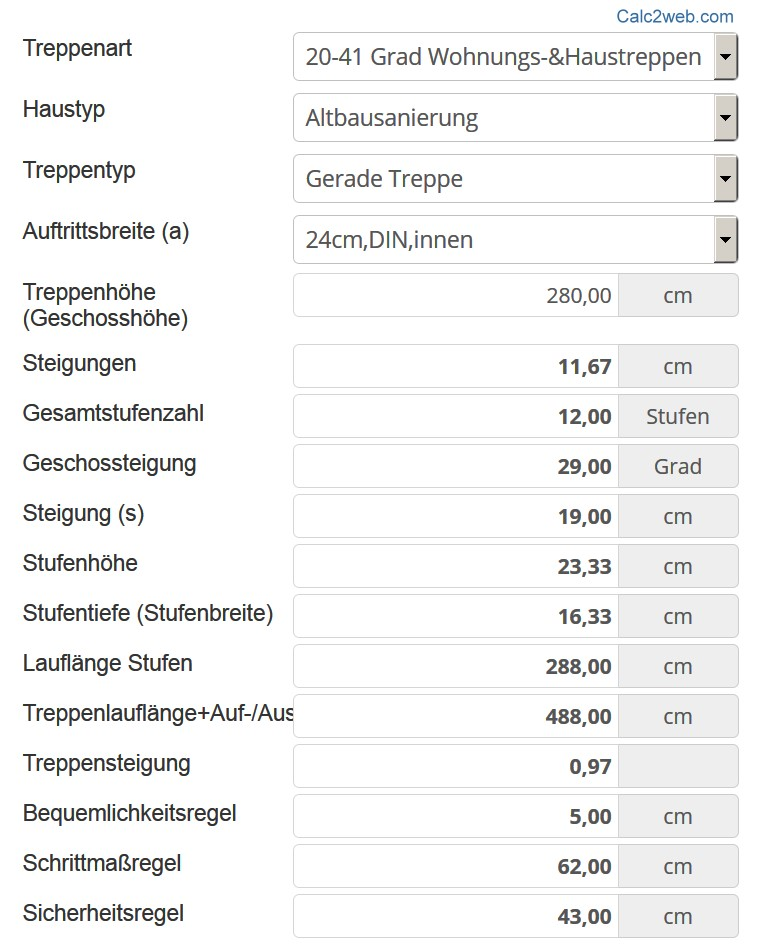# Price calculator stairs calculation - Calculate Now

 Type of stairs steepness Please select less than 6 degrees driveways, ramps 6-20 degrees: open stairs (outside) 21-30 degrees: public areas 20-41 degrees: apartment & house stairs 41-45 degrees: basement stairs > 45 degrees: steep stairs, ladders Type of house Please select New building Old building renovation Type of stairs Please select Straight staircases Step width (a) Please select flat:23cm,DIN,inside 24cm,DIN,inside 25cm,DIN,inside 26cm,DIN,inside steep:27cm,DIN,inside cm Stair height (floor height) cm cm Stairs width cm Gradients cm Total number of stages Stages Stufen Floor pitch Degrees Grad Gradient(s) cm cm Step height cm cm Step depth (step width) cm cm Running length steps cm cm Staircase runner+rise/fall cm cm Stair rise Convenience rule cm cm Step size rule cm cm Safety rule cm cm Kopffreiheit 0 cm >=200 DIN

## How to calculate the dimensions of a staircaseWhen building a new house, stairs must also be planned. It is clear that the steeper the staircase is designed, the less space it requires. However, the risk of accidents is also higher. In order to be able to plan a safe staircase, you should know some basic values, such as the optimum width of a step or the step size. Various formulas are available to calculate values such as

• running length,

• number of steps,

• riser height or

to calculate.

## Calculate stair dimensions - you should know these formulasFor a straight staircase, the run length can be easily calculated by tread width and number of steps. The formula is

The number of treads is also quite simple to calculate. You need the height of the floor and the desired riser height for one step. This results in the following formula:

Number of treads = floor height/pitch height.

The riser height in turn results from the calculated number of steps. The exact riser height is calculated using the following formula:

Exact riser height = floor height/number of treads.

Dimensions between 16 and 18 centimeters have proven to be the optimum step height for comfortable ascending and descending. This leaves the tread width, which reflects the depth of the individual steps. In practice, values of 29 centimeters have proven themselves. For the calculation, the step dimension is needed, which for people of average height is 0.63 meters. The formula is therefore as follows:

Step width = 63 centimeters - 2 x rise height.

Last but not least, it is necessary to calculate the slope of the stairs. Here it should be noted that the determined value does not exceed 1 and does not fall below 0.45, in order to be able to climb up and down the stairs as safely as possible and not to waste space unnecessarily. The formula for this is:

Slope = floor height/running length

If this was too many formulas for you, which only lead to the optimum staircase dimensions through repeated trial and error, you can also use our online calculator to calculate the staircase dimensions.

##### Similar Calculator Topics: Building & living, interior design